# Selina Solutions Concise Maths Class 7 Chapter 11: Fundamental Concepts (Including Fundamental Operations) Exercise 11D

Selina Solutions Concise Maths Class 7 Chapter 11 Fundamental Concepts (Including Fundamental Operations) Exercise 11D provides students with an in-depth knowledge about the concepts. Exercise 11D has problems on division of monomial and polynomial functions, according to the textbook prescribed by the ICSE board. The solutions have steps which can be followed in solving the problems, with ease. To perform well in the annual exam, Selina Solutions Concise Maths Class 7 Chapter 11 Fundamental Concepts (Including Fundamental Operations) Exercise 11D PDF links, which are provided below for free download.

## Selina Solutions Concise Maths Class 7 Chapter 11: Fundamental Concepts (Including Fundamental Operations) Exercise 11D Download PDF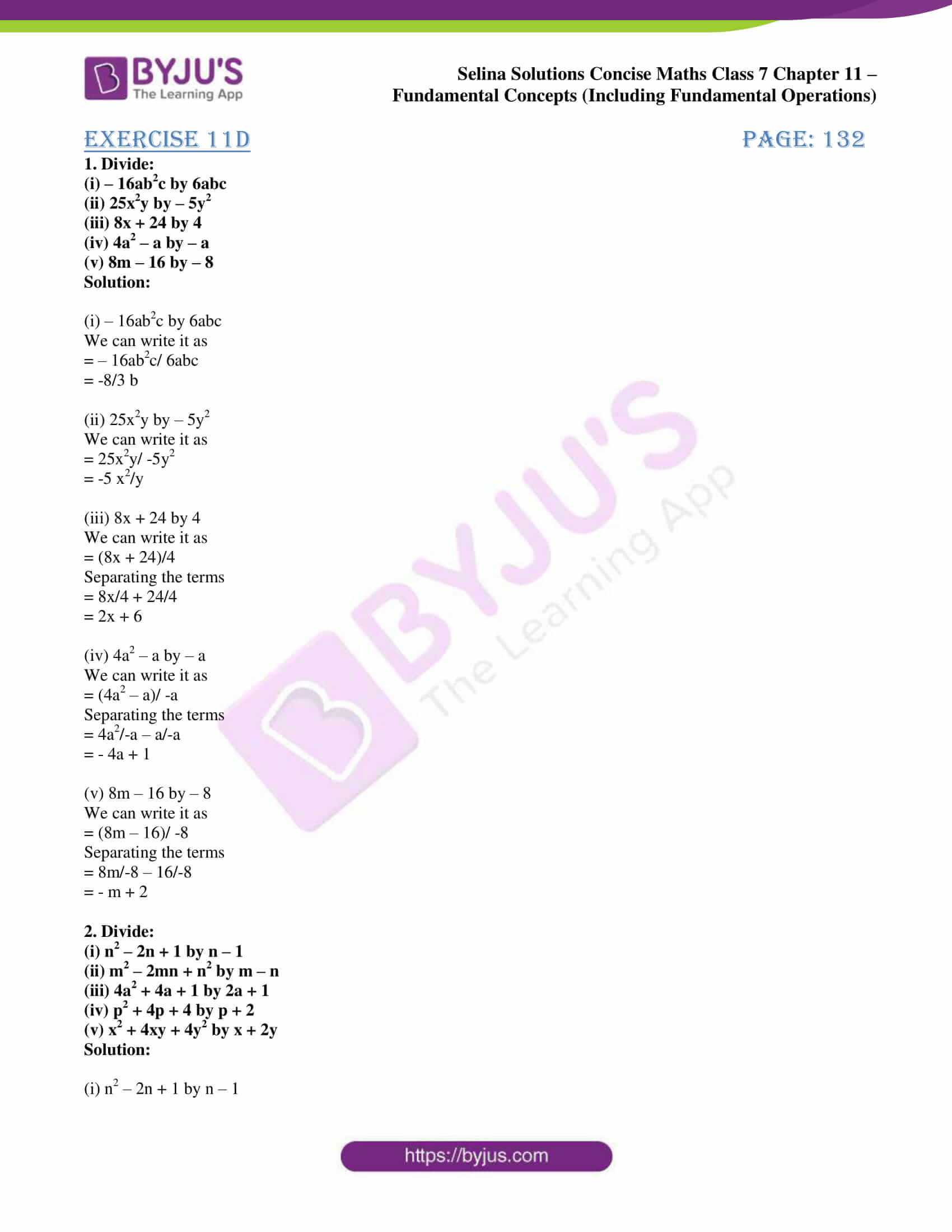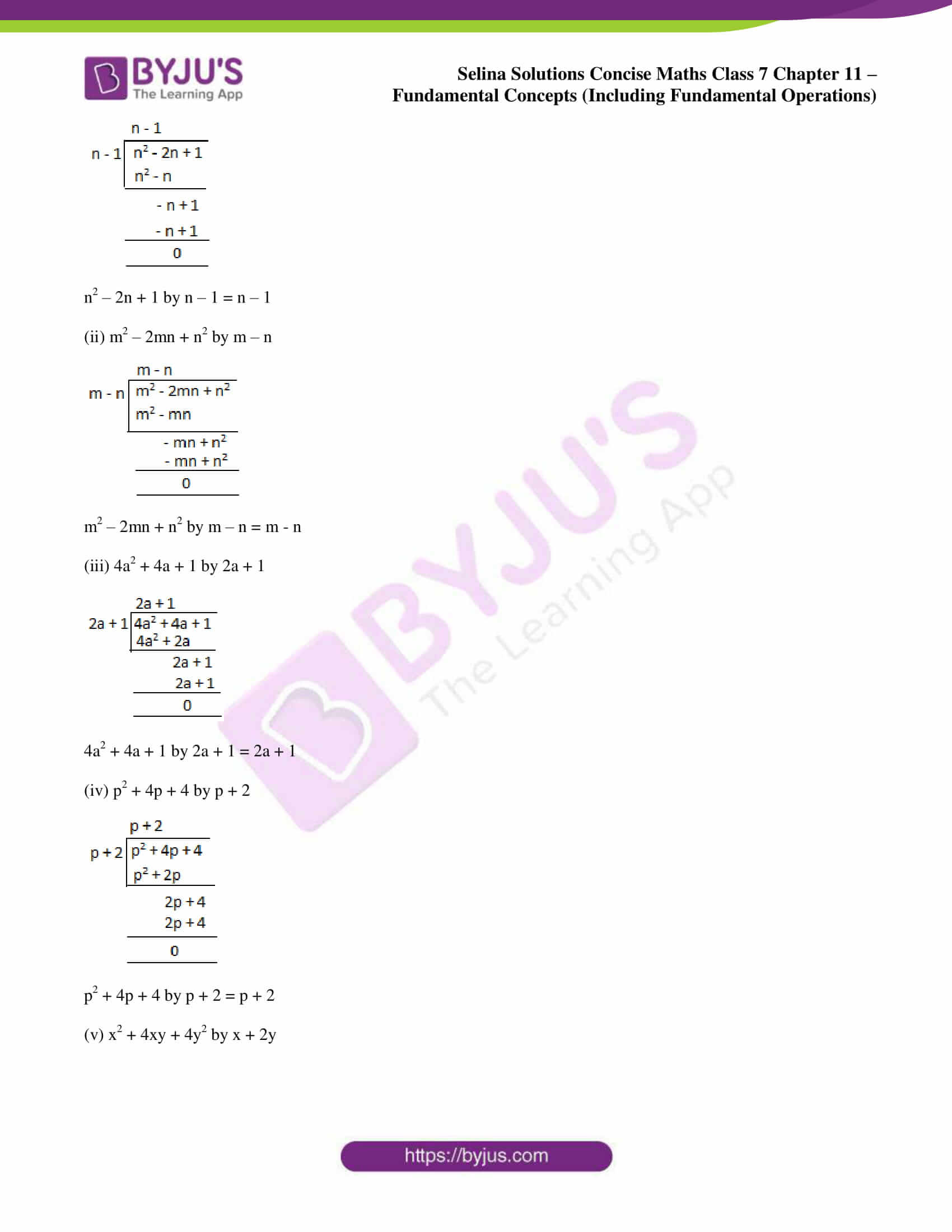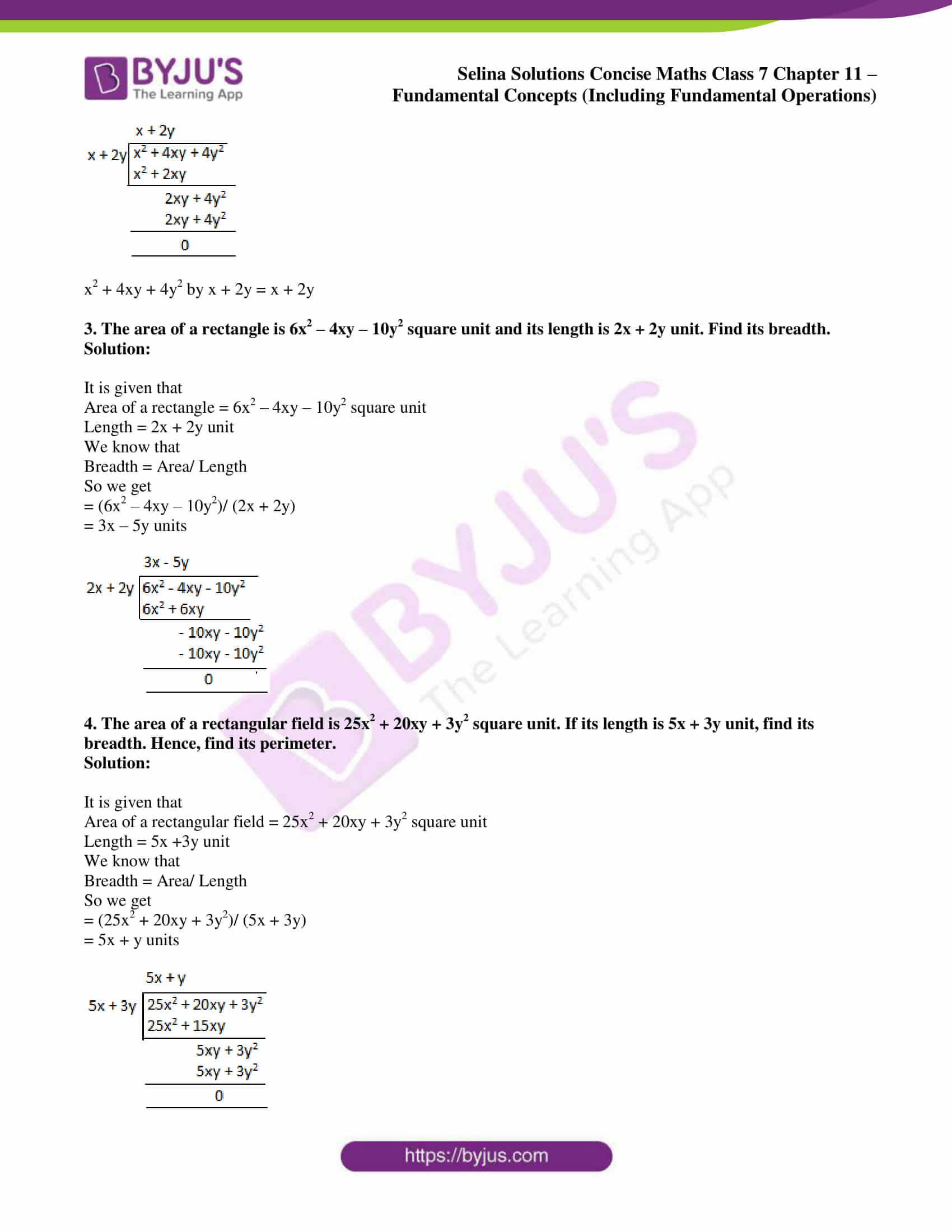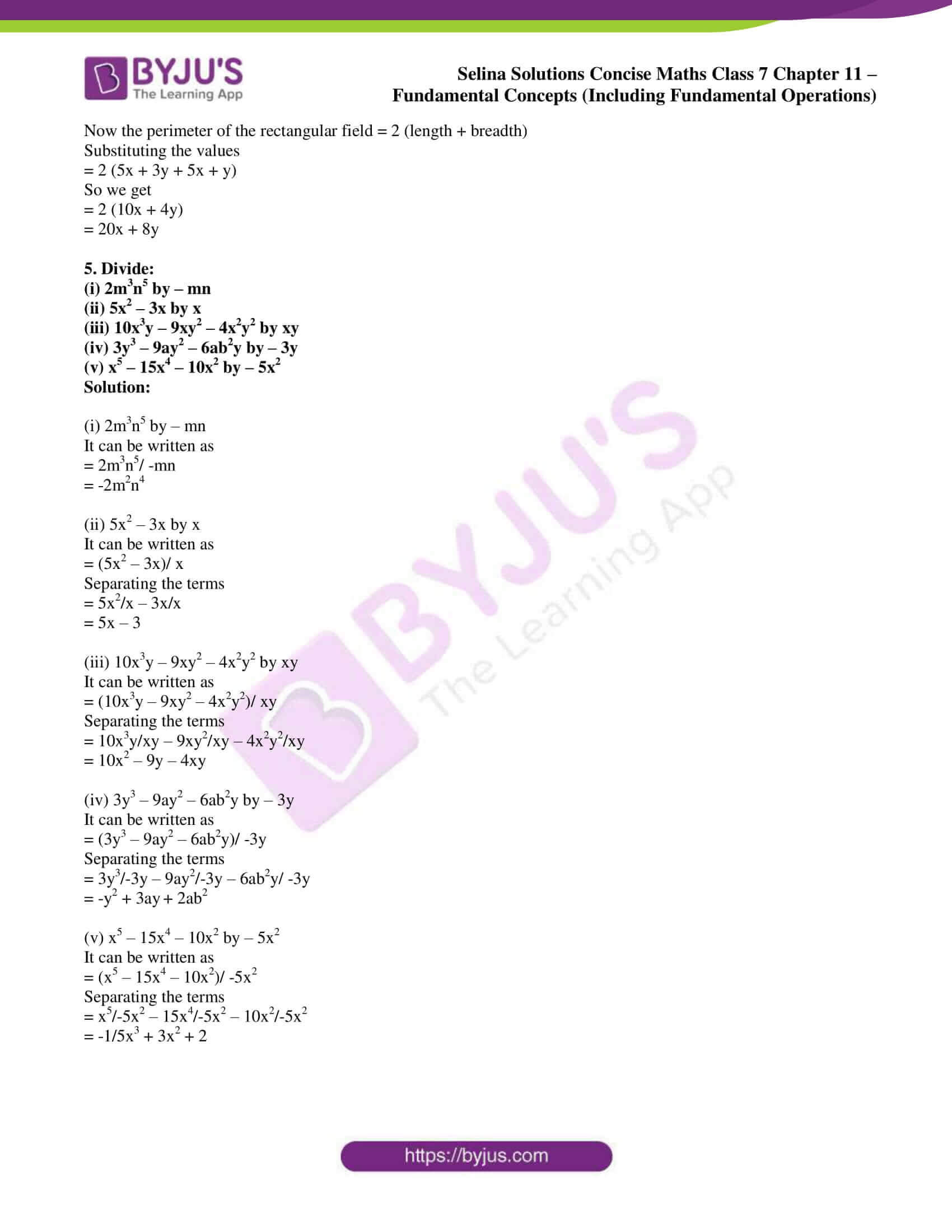### Access other exercises of Selina Solutions Concise Maths Class 7 Chapter 11: Fundamental Concepts (Including Fundamental Operations)

Exercise 11A Solutions

Exercise 11B Solutions

Exercise 11C Solutions

Exercise 11E Solutions

Exercise 11F Solutions

### Access Selina Solutions Concise Maths Class 7 Chapter 11: Fundamental Concepts (Including Fundamental Operations) Exercise 11D

#### Exercise 11D page: 132

1. Divide:

(i) – 16ab2c by 6abc

(ii) 25x2y by – 5y2

(iii) 8x + 24 by 4

(iv) 4a2 – a by – a

(v) 8m – 16 by – 8

Solution:

(i) – 16ab2c by 6abc

We can write it as

= – 16ab2c/ 6abc

= -8/3 b

(ii) 25x2y by – 5y2

We can write it as

= 25x2y/ -5y2

= -5 x2/y

(iii) 8x + 24 by 4

We can write it as

= (8x + 24)/4

Separating the terms

= 8x/4 + 24/4

= 2x + 6

(iv) 4a2 – a by – a

We can write it as

= (4a2 – a)/ -a

Separating the terms

= 4a2/-a – a/-a

= – 4a + 1

(v) 8m – 16 by – 8

We can write it as

= (8m – 16)/ -8

Separating the terms

= 8m/-8 – 16/-8

= – m + 2

2. Divide:

(i) n2 – 2n + 1 by n – 1

(ii) m2 – 2mn + n2 by m – n

(iii) 4a2 + 4a + 1 by 2a + 1

(iv) p2 + 4p + 4 by p + 2

(v) x2 + 4xy + 4y2 by x + 2y

Solution:

(i) n2 – 2n + 1 by n – 1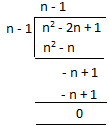n2 – 2n + 1 by n – 1 = n – 1

(ii) m2 – 2mn + n2 by m – n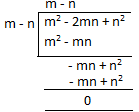m2 – 2mn + n2 by m – n = m – n

(iii) 4a2 + 4a + 1 by 2a + 1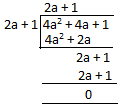4a2 + 4a + 1 by 2a + 1 = 2a + 1

(iv) p2 + 4p + 4 by p + 2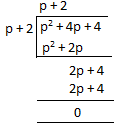p2 + 4p + 4 by p + 2 = p + 2

(v) x2 + 4xy + 4y2 by x + 2y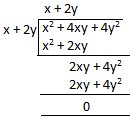x2 + 4xy + 4y2 by x + 2y = x + 2y

3. The area of a rectangle is 6x2 – 4xy – 10y2 square unit and its length is 2x + 2y unit. Find its breadth.

Solution:

It is given that

Area of a rectangle = 6x2 – 4xy – 10y2 square unit

Length = 2x + 2y unit

We know that

So we get

= (6x2 – 4xy – 10y2)/ (2x + 2y)

= 3x – 5y units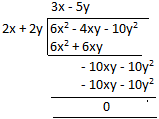4. The area of a rectangular field is 25x2 + 20xy + 3y2 square unit. If its length is 5x + 3y unit, find its breadth. Hence, find its perimeter.

Solution:

It is given that

Area of a rectangular field = 25x2 + 20xy + 3y2 square unit

Length = 5x +3y unit

We know that

So we get

= (25x2 + 20xy + 3y2)/ (5x + 3y)

= 5x + y units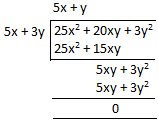Now the perimeter of the rectangular field = 2 (length + breadth)

Substituting the values

= 2 (5x + 3y + 5x + y)

So we get

= 2 (10x + 4y)

= 20x + 8y

5. Divide:

(i) 2m3n5 by – mn

(ii) 5x2 – 3x by x

(iii) 10x3y – 9xy2 – 4x2y2 by xy

(iv) 3y3 – 9ay2 – 6ab2y by – 3y

(v) x5 – 15x4 – 10x2 by – 5x2

Solution:

(i) 2m3n5 by – mn

It can be written as

= 2m3n5/ -mn

= -2m2n4

(ii) 5x2 – 3x by x

It can be written as

= (5x2 – 3x)/ x

Separating the terms

= 5x2/x – 3x/x

= 5x – 3

(iii) 10x3y – 9xy2 – 4x2y2 by xy

It can be written as

= (10x3y – 9xy2 – 4x2y2)/ xy

Separating the terms

= 10x3y/xy – 9xy2/xy – 4x2y2/xy

= 10x2 – 9y – 4xy

(iv) 3y3 – 9ay2 – 6ab2y by – 3y

It can be written as

= (3y3 – 9ay2 – 6ab2y)/ -3y

Separating the terms

= 3y3/-3y – 9ay2/-3y – 6ab2y/ -3y

= -y2 + 3ay + 2ab2

(v) x5 – 15x4 – 10x2 by – 5x2

It can be written as

= (x5 – 15x4 – 10x2)/ -5x2

Separating the terms

= x5/-5x2 – 15x4/-5x2 – 10x2/-5x2

= -1/5x3 + 3x2 + 2